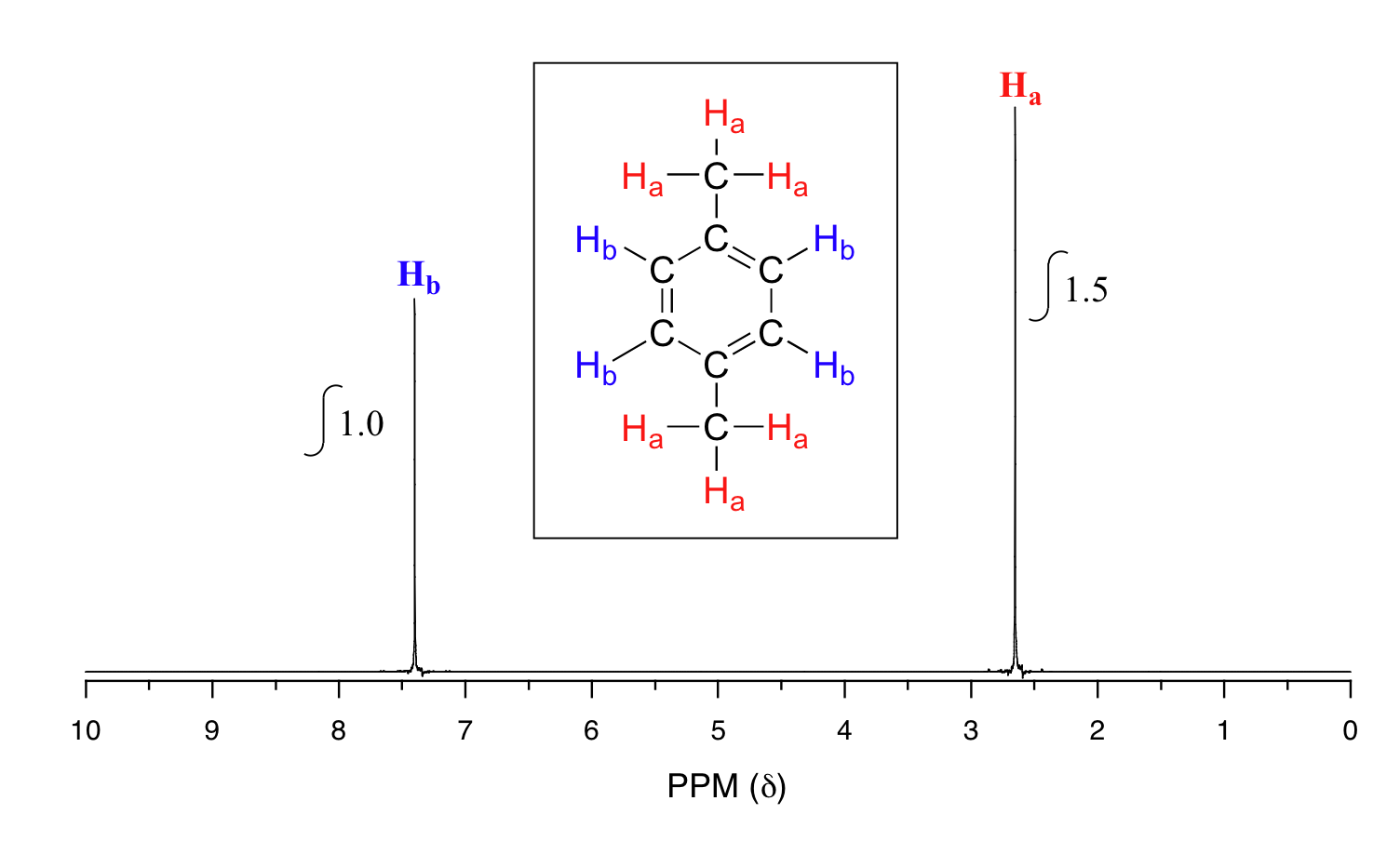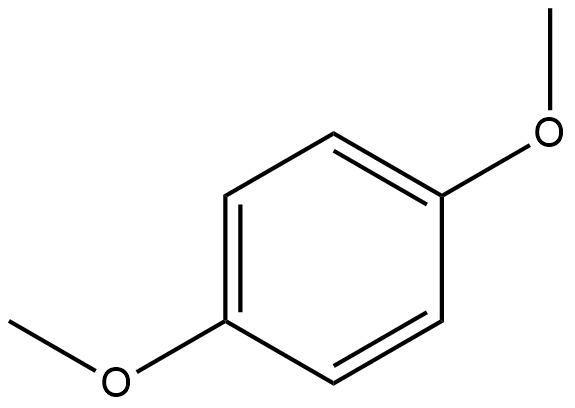## Integration of Proton NMR Absorptions

### Objectives

After completing this section, you should be able to

1. explain what information can be obtained from an integrated 1H NMR spectrum, and use this information in the interpretation of such a spectrum.
2. use an integrated 1H NMR spectrum to determine the ratio of the different types of protons present in an organic compound.

### Study Notes

The concept of peak integration is that the area of a given peak in a 1H NMR spectrum is proportional to the number of (equivalent) protons giving rise to the peak. Thus, a peak which is caused by a single, unique proton has an area which measures one third of the area of a peak resulting from a methyl (CH3) group in the same spectrum.

In practice, we do not have to measure these areas ourselves: it is all done electronically by the spectrometer, and an integration curve is superimposed on the rest of the spectrum. The integration curve appears as a series of steps, with the height of each step being proportional to the area of the corresponding absorption peak, and consequently, to the number of protons responsible for the absorption.

As it can be difficult to decide precisely where to start and stop when measuring integrations, you should not expect your ratios to be exact whole numbers.

### Signal integration

The computer in an NMR instrument can be instructed to automatically integrate the area under a signal or group of signals.  This is very useful, because in 1H-NMR spectroscopy the area under a signal is proportional to the number of hydrogens to which the peak corresponds.  The two signals in the methyl acetate spectrum, for example, integrate to approximately the same area, because they both correspond to a set of three equivalent protons.

Take a look next at the spectrum of para-xylene  (IUPAC name 1,4-dimethylbenzene):This molecule has two sets of protons: the six methyl (Ha) protons and the four aromatic (Hb) protons.  When we instruct the instrument to integrate the areas under the two signals, we find that the area under the peak at 2.6 ppm is 1.5 times greater than the area under the peak at 7.4 ppm. This (along with the actual chemical shift values, which we’ll discuss soon) tells us which set of protons corresponds to which NMR signal.

The integration function can also be used to determine the relative amounts of two or more compounds in a mixed sample.  If we have a sample that is a 50:50 (mole/mole) mixture of benzene and acetone, for example, the acetone signal should integrate to the same value as the benzene sample, because both signals represent six equivalent protons.  If we have a 50:50 mixture of acetone and cyclopentane, on the other hand, the ratio of the acetone peak area to the cylopentane peak area will be 3:5 (or 6:10), because the cyclopentane signal represents ten protons.

### Example

You take a 1H-NMR spectrum of a mixed sample of acetone (CH3(CO)CH3) and dichloromethane (CH2Cl2).  The integral ratio of the two signals (acetone : dichloromethane) is 2.3 to 1.  What is the molar ratio of the two compounds in the sample?

### Example

You take the 1H-NMR spectrum of a mixed sample of 36%  para-xylene and 64% acetone in CDCl3 solvent (structures are shown earlier in this chapter).  How many peaks do you expect to see?  What is the expected ratio of integration values for these peaks? (set the acetone peak integration equal to 1.0)

### Exercise

#### Question

Predict how many signals the following molecule would have? Sketch the spectra and estimate the integration of the peaks.